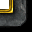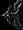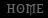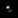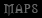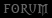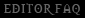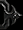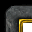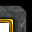Tutorial By Nestharus

• What is a Save/Load code?
• Compression
[list=1]
• Value Replacement
• Placeholders
• Base Conversion
• Professional Information Storage
• Data Representation

• Checksums
• Overflow/Underflow Detection
• Encryption
[/list]

A save/load code is a way to save player information online. Warcraft 3 has a useful thing called a gamecache, which is used to save player data; however, it only works in single player mode. This means that if it was used in multiplayer mode, it would not load from one game to another because it wouldn't write to the actual hard drive. To resolve this, maps use codes: strings that contain information concerning the player's character. The data is outputted to the player and then the player types it into another game to get their character back. The string contains encrypted information that is unreadable to the player but readable to the save/load system in the map.

Save/load codes can store many values. For example, it may store the id of the player's hero, six items in the inventory, the level of the hero, the xp of the hero, and the stats of the hero (strength, agility, and intelligence).

A simple collection of data might be-

• Item 1: [ljass]'afac'[/ljass] (Alleria's Flute of Accuracy)
• Item 2: [ljass]'spsh'[/ljass] (Amulet of Spell Shield)
• Item 3: [ljass]'ajen'[/ljass] (Ancient Janggo of Endurance)
• Item 4: [ljass]'bspd'[/ljass] (Boots of Speed)
• Item 5: [ljass]'bgst'[/ljass] (Belt of Giant Strength +6)
• Item 6: [ljass]'belv'[/ljass] (Boots of Quel'Thalas +6)
• xp: [ljass]1200[/ljass] (level doesn't need to be stored with xp)
• strength: [ljass]44[/ljass]
• agility: [ljass]22[/ljass]
• intelligence: [ljass]33[/ljass]

Recall that object type ids (like unit type ids and item types ids) are stored in ascii (ascii table of 256). This means that the values, like Hpal, are really something else entirely. If a user were to type Hpal in as input, the value would be a string ("Hpal", not 'Hpal').

As actual numbers, the data would be

• Item 1: [ljass]1634099555[/ljass] (Alleria's Flute of Accuracy)
• Item 2: [ljass]1936749416[/ljass] (Amulet of Spell Shield)
• Item 3: [ljass]1634362734[/ljass] (Ancient Janggo of Endurance)
• Item 4: [ljass]1651732580[/ljass] (Boots of Speed)
• Item 5: [ljass]1650946932[/ljass] (Belt of Giant Strength +6)
• Item 6: [ljass]1650814070[/ljass] (Boots of Quel'Thalas +6)
• xp: [ljass]1200[/ljass] (level doesn't need to be stored with xp)
• strength: [ljass]44[/ljass]
• agility: [ljass]22[/ljass]
• intelligence: [ljass]33[/ljass]

The output code would be-
[indent]
Player View
-> [ljass]1215324524-1634099555-1936749416-1634362734-1651732580-1650946932-1650814070-1200-44-22-33[/ljass]

Map View
-> [ljass]1215324524-1634099555-1936749416-1634362734-1651732580-1650946932-1650814070-1200-44-22-33[/ljass]

The first value would be the hero. The next six values would be items. The eighth value would be the xp. The final three values would be strength, agility, and intelligence. The dashes in the code signify where one value begins and another one ends. While this is rather straight forward, easy to develop, and easy for the map to interpret, it outputs absolutely enormous codes (80 characters with 10 dashes) and is very easy to read by the player, making it easy to modify and possibly cheat.
[/indent]

Compression

The most important thing in save/load is the compression of data. Compression makes codes smaller, which makes it easier for players to retrieve those codes.

[point]value replacement[/point]
The best way to compress data is by using value replacement. A big number like 1215324524, which represents the Paladin unit type id, can be replaced by a value like 1. This value replacement is done through the use of catalogs.

Early catalogs were essentially arrays of data. When something was added to a catalog, it was given a unique catalog id.
[indent]
```			set catalog = 'Hpal'		//paladin
set catalog = 'Hmkg'		//mountain king```

In this case, the catalog would have two heroes in it. 'Hpal' is assigned id 1 and 'Hmkg' is assigned id 2.

The array can be used to easily convert catalog ids into unit type ids. If a catalog were to be read using the id of 1, 'Hpal' would be returned. This means that in loading, when reading values back out like 1, they would be passed into the catalog to retrieve the actual values -> catalog.

```			local integer heroId = hero id from code //( 1 )

set heroId = catalog[heroId] //retrieve actual value of hero id```

To convert unit type ids into catalog ids, a search was required. The array would be looped through until the unit type id was found or the catalog was looped over in its entirety.

```			local integer value = GetUnitTypeId(unitBeingSaved) //( \'Hpal\' )

local integer id = 1								//first id of catalog

loop
exitwhen catalog[id] == value or catalog[id] == 0
set id = id + 1
endloop```

In the case where the value catalog[id] is zero, the searched for unit type id was not in the catalog.

When Blizzard implemented hashtables, the search method was abandoned. With hashtables, the catalog id could be retrieved using the unit type id.

```			call SaveInteger(catalogTable, 'Hpal', 0, 1)
call SaveInteger(catalogTable, 'Hmkg', 0, 2)```

As such, all catalogs have two parts to them: an array part and a hashtable part. The array part is responsible for converting catalog ids into unit type ids. The hashtable part is responsible for converting unit type ids into catalog ids.

```			catalog[id] -> unitTypeId

The Catalog resource is used for save/load catalogs.

The previous data is now compressed through the use of two catalogs, one for heroes and one for items.

```			struct HeroCatalog extends array
implement Catalog

private static method onInit takes nothing returns nothing
endmethod
endstruct```

```			struct ItemCatalog extends array
implement Catalog

private static method onInit takes nothing returns nothing
call add('afac')		//id of 1	(Alleria\'s Flute of Accuracy)
call add('spsh')		//id of 2	(Amulet of Spell Shield)
call add('ajen')		//id of 3	(Ancient Janggo of Endurance)
call add('bspd')		//id of 4	(Boots of Speed)
call add('bgst')		//id of 5	(Belt of Giant Strength +6)
call add('belv')		//id of 6	(Boots of Quel\'Thalas +6)
endmethod
endstruct```

The values can now be converted to catalog ids and back to their original values

```			HeroCatalog['Hpal'].id	-> 1
HeroCatalog.raw -> 'Hpal'```

The data is now converted into catalog ids

```			HeroCatalog['Hpal'].id	-> 1		//(Paladin)

ItemCatalog['afac'].id	-> 1		//(Alleria\'s Flute of Accuracy)
ItemCatalog['spsh'].id	-> 2		//(Amulet of Spell Shield)
ItemCatalog['ajen'].id	-> 3		//(Ancient Janggo of Endurance)
ItemCatalog['bspd'].id	-> 4		//(Boots of Speed)
ItemCatalog['bgst'].id	-> 5		//(Belt of Giant Strength +6)
ItemCatalog['belv'].id	-> 6		//(Boots of Quel\'Thalas +6)```

The converted data is as follows

• Item 1: [ljass]1[/ljass] (Alleria's Flute of Accuracy)
• Item 2: [ljass]2[/ljass] (Amulet of Spell Shield)
• Item 3: [ljass]3[/ljass] (Ancient Janggo of Endurance)
• Item 4: [ljass]4[/ljass] (Boots of Speed)
• Item 5: [ljass]5[/ljass] (Belt of Giant Strength +6)
• Item 6: [ljass]6[/ljass] (Boots of Quel'Thalas +6)
• xp: [ljass]1200[/ljass] (level doesn't need to be stored with xp)
• strength: [ljass]44[/ljass]
• agility: [ljass]22[/ljass]
• intelligence: [ljass]33[/ljass]

The resulting code is

Player View
-> [ljass]1-1-2-3-4-5-6-1200-44-22-33[/ljass]

Map View
-> [ljass]1-1-2-3-4-5-6-1200-44-22-33[/ljass]

The code is now 17 characters long with 10 dashes, which is much improved over 80 characters with 10 dashes. It is still easy to read by the map. However, it is still also easy to read by players.

[point]placeholder[/point]
The next way to compress data is to remove the dashes. Right now, the dashes mark where each value begins and ends. Removing the dashes requires that the map needs to know when each value begins and ends without the use of the dashes. By telling the map how big each number should be, it can figure out where each value begins and ends.

Each number takes up a certain number of space, this space being that number's amount of digits. For example, 1200 has four digits. By knowing the maximum value a number can be, the number of digits required to store that number in a code can be calculated.

If a map has a max level of 10, then the maximum xp value (assuming default settings) is 5400. The value 5400 has 4 digits in it, meaning that the code needs to have 4 digits reserved for the xp value.

Storing 1200
-> [ljass]1200[/ljass]

Storing 5400
-> [ljass]5400[/ljass]

Store 60
-> [ljass]0060[/ljass]

Store 0
-> [ljass]0000[/ljass]

A number can't just be thrown into the code as it may then have variable size. For example, the value 60 only has 2 digits. A maximum value of 5400 has 4 digits. If a position can have between 2 and 4 digits, the map has no way of knowing exactly how big the number is, meaning that it won't be able to read it out of the code. The maximum value must always be used and all of the digits required to store that value must always be reserved, even if they all turn out to be 0.

Determine the maxes

• Hero max: [ljass]1[/ljass]
• Item max: [ljass]6[/ljass] (Note that all 6 items share the same max)
• xp max: [ljass]5400[/ljass] (Level 10 is 5400 xp)
• Stat max [ljass]256[/ljass] (Note that all 3 stats share the same max)

Count the number of digits in each max

• Hero max: [ljass]1[/ljass] digit
• Item max: [ljass]1[/ljass] digit
• xp max: [ljass]4[/ljass] digits
• Stat max [ljass]3[/ljass] digits

This results in a new code

Player View
-> [ljass]11234561200044022033[/ljass]

Map View
-> [ljass]1-1-2-3-4-5-6-1200-044-022-033[/ljass]

The map knows when one value ends and one value begins because it knows exactly how big each position is. The first position in the code will always have exactly 1 digit. The last value in the code will always have exactly three digits.

This new code is 20 characters long with 0 dashes. This is slightly longer than the 17 character code, but it is shorter than it when accounting for the dashes (20 vs 27). However, the problem with this is that the code is now hard to read. Dashes now need to be added in for readability. These dashes are now completely ignored by the map and are just there so that the player can read the code.

Player view
-> [ljass]1123-4561-2000-4402-2033[/ljass]

Map view
-> [ljass]1-1-2-3-4-5-6-1200-044-022-033[/ljass]

The final code size, including the dashes, is 24 characters.

A look at the minimum and maximum code sizes

Minimum Code
-> [ljass]0000-0000-0000-0000-0000[/ljass]

Maximum Code
-> [ljass]1123-4565-4002-5625-6256[/ljass]

[point]base conversion[/point]
The code can still be compresed. Right now, the code is being outputted in the base 10 number system. Base 10 means that there are 10 symbols that can be used to represent a digit. Base 10 may only go up to 9, which may seem odd. The 10 in base 10 tells how many *symbols* there are in the base, not what the biggest symbol is (in this case 9). Base 10 uses 0123456789.

Counting

1,2,3,4,5,6,7,8,9,10
0,1,2,3,4,5,6,7,8,9

Note that there are 10 symbols in base 10. The more symbols that there are in a base, the smaller the number of digits are required to represent a value. The actual formula for calculating the number of digits required to represent a value is log(value)/log(base)+1.

To represent 1000 in base 10, it would require 4 digits. This can be calculated by either counting out the digits in 1000 or by calculating log(1000)/log(10)+1, which is 4.

1000 represented in base 2 would require 10 digits (10.966). log(1000)/log(2)+1 = 10.966. Base 2, or binary, uses the digits 0 and 1.

1000 in Base 2
-> [ljass]1111101000[/ljass]

The larger the base, the smaller the number of digits to represent a value is. For example, base 62 (a-z, A-Z, 0-9) can represent 1000 with 2 digits (log(1000)/log(62)+1 = 2).

The most common base used to compress data in save/load is alpha-numeric, or 0123456789abcdefghijklmnopqrstuvwxyzABCDEFGHIJKLMNOPQRSTUVWXYZ. Converting numbers to different bases is done with Big Int.

After commpressing the data by converting the bases, it as follows-

• Item 1: [ljass]1[/ljass] (Alleria's Flute of Accuracy)
• Item 2: [ljass]2[/ljass] (Amulet of Spell Shield)
• Item 3: [ljass]3[/ljass] (Ancient Janggo of Endurance)
• Item 4: [ljass]4[/ljass] (Boots of Speed)
• Item 5: [ljass]5[/ljass] (Belt of Giant Strength +6)
• Item 6: [ljass]6[/ljass] (Boots of Quel'Thalas +6)
• xp: [ljass]jm[/ljass]
• strength: [ljass]I[/ljass]
• agility: [ljass]m[/ljass]
• intelligence: [ljass]x[/ljass]

The maximum values converted to base 62 are as follows

• Hero max: [ljass]1[/ljass] (1)
• Item max: [ljass]6[/ljass] (6)
• xp max: [ljass]1p6[/ljass] (5400)
• Stat max [ljass]48[/ljass] (256)

The new maximum digits required are as follows

• Hero max: [ljass]1[/ljass] (1 has 1 digit in it)
• Item max: [ljass]1[/ljass] (6 has 1 digit in it)
• xp max: [ljass]3[/ljass] (lp6 has 3 digits in it)
• Stat max [ljass]2[/ljass] (48 has 2 digits in it)

The resulting code

Player View
-> [ljass]1123-4560-jm0I-0m0x[/ljass]

Map View
-> [ljass]1-1-2-3-4-5-6-0jm-0I-0m-0x[/ljass]

-> [ljass]0000-0000-0000-0000[/ljass]

-> [ljass]1666-666l-p648-4848[/ljass]

The code is now 16 characters long with 3 dashes, a bit improved over 20 characters long with 4 dashes.

[point]pro info storage[/point]
The next method used to compress data is to store the numbers in different bases. Right now, all of the numbers are stored in one base, this base being base 62. The base states exactly how many digits are required to represent a number. Base 62 was chosen since it is very readable and it requires a smaller amount of digits than base 10.

Each number in a code can be represented in its own base, and these bases can be chosen arbitrarily. This means that a number can be stored in a base that represents it in exactly 1 digit.

Looking back at the max values

• Hero max: [ljass]1[/ljass]
• Item max: [ljass]6[/ljass]
• xp max: [ljass]5400[/ljass]
• Stat max [ljass]256[/ljass]

The hero max can be stored in base 2, the item max in base 7, the xp max in base 5401, and the stat max in base 257. Remember again, the base isn't how big a digit can go (base 10 only goes to 9), it is the number of symbols in that base (base 10 has 10 symbols). So to store a max value of 5400 in exactly 1 digit, base 5401 is needed, not base 5400.

By storing numbers in their own bases, the map view changes

[ljass]2-7-7-7-7-7-7-5401-257-257-257[/ljass]

Rather than seeing the values inside of a code, the map now sees the bases used in a code. It may seem to be rather bad compared to previous methods as the values it is looking at are larger than even the maximal values for the code, but this is not the case.

Recall that in base 62, each digit can go up to a value of 61. If the hero, which has a maximum value of 1, is stored in exactly 1 digit, then it is stored with a maximum possible value of 61, not 1.

The amount of information stored in the digit counting scheme with base 62

[ljass]62-62-62-62-62-62-62-238328-3844-3844-3844[/ljass]

Notice how much larger the slots are in the digit counting scheme. From here, it can be seen that the code resulting from storing each value in its own base is going to be much smaller than the code resulting from storing all of the numbers in one base (like base 62).

To add a digit to a number in a given base, it is number*base+digit. In base 10, if there was a number 567 and the digit 8 were to be added to it, it would turn into 5678. To do this mathematically, it would be 567*10+8.

[ljass]567*10 = 5670 + 8 = 5678[/ljass]

Notice that when the number is multiplied by a base, exactly 1 digit is added for that base. From there, a value can be stored into that digit. Also, a number with different digit sizes in it can't be read by a user, but it can be read by a map.

Data

• Item 1: [ljass]1[/ljass] (Alleria's Flute of Accuracy)
• Item 2: [ljass]2[/ljass] (Amulet of Spell Shield)
• Item 3: [ljass]3[/ljass] (Ancient Janggo of Endurance)
• Item 4: [ljass]4[/ljass] (Boots of Speed)
• Item 5: [ljass]5[/ljass] (Belt of Giant Strength +6)
• Item 6: [ljass]6[/ljass] (Boots of Quel'Thalas +6)
• xp: [ljass]1200[/ljass]
• strength: [ljass]44[/ljass]
• agility: [ljass]22[/ljass]
• intelligence: [ljass]33[/ljass]

The require bases for the code are again 2, 7, 5401, and 257. The number always starts at 0 (with nothing in it).

Add a base 2 digit to the number for the hero

[ljass]0 * 2 = 0[/ljass]

[ljass]0 + 1 = 1[/ljass]

Add a base 7 digit to the number for item 1

[ljass]1 * 7 = 7[/ljass]

Add the item 1 value to the new digit

[ljass]7 + 1 = 8[/ljass]

```			8 * 7 = 56			(item 2 digit)
56 + 2 = 58			(item 2 value)

58 * 7 = 406		(item 3 digit)
406 + 3 = 409		(item 3 vaule)

409 * 7 = 2863		(item 4 digit)
2863 + 4 = 2867		(item 4 value)

2867 * 7 = 20069	(item 5 digit)
20069 + 5 = 20074	(item 5 value)

20074 * 7 = 140518	(item 6 digit)
140518 + 6 = 140524	(item 6 value)```

Add a base 5401 digit to the number for xp

[ljass]140524 * 5401 = 758970124[/ljass]

Add the xp value to the new digit

[ljass]758970124 + 1200 = 758971324[/ljass]

Add a base 257 digit to the number for strength

[ljass]758971324 * 257 = 195055630268[/ljass]

Add strength value to new digit

[ljass]195055630268 + 44 = 195055630312[/ljass]

```			195055630312 * 257 = 50129296990184			(agility digit)
50129296990184 + 22 = 50129296990206		(agility value)
50129296990206 * 257 = 12883229326482942	(intelligence digit)
12883229326482942 + 33 = 12883229326482975	(intelligence value)```

The resulting value is 12883229326482975. 2^31-1, or 2147483647, is the maximum value that can fit into an integer. 12883229326482975 is way above that maximum value. One reason BigInt is required for good save/load is because it can store values of up to 8191 digits regardless of the base. 12883229326482975 is only 17 digits, meaning that it can easily fit into a BigInt.

The final code in base 10

Player View
-> [ljass]1288-3229-3264-8297-5[/ljass]

Map view
-> [ljass]2-7-7-7-7-7-7-5401-257-257-257[/ljass]

Notice that the map can't see the actual values in the code now. However, it does know the structure of the code, so it can retrieve the values out of the code.

The logarithm equation can be used to calculate the number of bits required for a code

[ljass]log(2)/log(10) + log(7)/log(10)*6 + log(5401)/log(10) + log(257)/log(10)*3 + 1 = 17.33 digits[/ljass]

The final step is to convert the code into the target base, which in this case is base 62.

Player View
-> [ljass]X0kt-zun4-H[/ljass]

Map view
-> [ljass]2-7-7-7-7-7-7-5401-257-257-257[/ljass]

Digit calculation

[ljass]log(2)/log(62)+log(7)/log(62)*6+log(5401)/log(62)+log(257)/log(62)*3 = 9.11 digits[/ljass]

The code is now 9 digits long with 2 dashes, much improved over 16 digits long with 3 dashes. This almost 50% decrease in size was expected because the amount of information stored in the code is almost half.

Loading requires that the digits be read out of the code. For this, the bases have to be divided out.

If one were to take the first digit out of 5678, they would get 8 and be left with 567. Division is used to do this.

```			   567 r8
-----
10|5678
50
67
60
78
70
8```

The remainder is the retrieved digit and 567 is the what's left of the number.

In a calculator, this can be done by doing 5678/10 (remaining number) and 5678 Mod 10 (remainder). Ignore the decimals.

Dividing by the base retrieves the last added digit. This means that for one, loading must be done in the reverse order of saving. If strength, agility, and intelligence were saved in that order, intelligence, agility, and strength would have to be loaded in that order (backwards). For two, each digit must be divided out with the base that it is stored in.

Code in base 10

[ljass]12883229326482975[/ljass]

Maximum Values

• Hero max: [ljass]1[/ljass]
• Item max: [ljass]6[/ljass]
• xp max: [ljass]5400[/ljass]
• Stat max [ljass]256[/ljass]

The data must be loaded in this order

1. intelligence
2. agility
3. strength
4. xp
5. item 6
6. item 5
7. item 4
8. item 3
9. item 2
10. item 1
11. hero

Divide the number by the base that intelligence is stored in (base 257)

[ljass]12883229326482975/257 = 50129296990206 r33 //(intelligence is 33)[/ljass]

Retrieve agility and strength

```			50129296990206/257 = 195055630312 r22	//(agility is 22)
195055630312/257 = 758971324 r44		//(strength is 44)```

Divide the number by the base that xp is stored in (base 5401)

[ljass]758971324/5401 = 140524 r1200 //(xp is 1200)[/ljass]

Divide the number by the base that item 6 is store in (base 7)

[ljass]140524/7 = 20074 r6 //(item 6 is 6)[/ljass]

Retrieve the other five items

```			20074/7 = 2867 r5						//(item 5 is 5)
2867/7 = 409 r4							//(item 4 is 4)
409/7 = 58 r3							//(item 3 is 3)
58/7 = 8 r2								//(item 2 is 2)
8/7 = 1 r1								//(ietm 1 is 1)```

Retrieve the hero

[ljass]1/2 = 0 r1 //(hero is 1)[/ljass]

[point]data representation[/point]
The next way to compress data is by representing data in different ways. For example, xp may be represented as a percent (how close the hero is to leveling). x,y coordinates, facing, life, and mana may also be represented as percents.

If xp was stored as a percent, the level would also need to be stored. Rather than 1200, the values would be 4 and 60, 4 being the level and 60 being 60% into that level.

To retrieve percents of xp, Hero Reward is used. The reason that this must be used is because large levels may overflow. It also fixes a lot of other issues with Warcraft 3's leveling system. x, y, facing, life, and mana percents can be retrieved by using Unit State Percent.

After doing all of the math using the new values of 4 and 60 in place of 1200, the new code is 2623879390477381 in base 10.

Comparison

Code 1 in Base 10 -> [ljass]12883229326482975[/ljass]
Code 2 in Base 10 -> [ljass]2623879390477381[/ljass]

Code 1 in Base 62 -> [ljass]X0kt-zun4-H[/ljass]
Code 2 in Base 62 -> [ljass]c14R-OWXS-l[/ljass]

This last compression technique didn't result in less characters, but it still resulted in a smaller number, meaning that there is more room for new information without increasing the code size.

[point]conditional saving[/point]
The final compression technique is conditional saving. Rather than compression the data, condition saving only saves data that it thinks is important. For example, if the hero is at a maximum level of 10, then xp doesn't need to be saved.

This simply uses if statements to determine whether or not a value should be saved into a code.

```			if (GetHeroLevel(hero) < GetMaxLevel()) then
store xp
endif
store level```

The reason xp is stored before the level is so that the level can be read out of the code first. The code can't know whether the xp is in the code or not without the level. In conditional save/load, the order can really be important as values are dependent on other values saved into the code (in this case the level of the hero).

This uses an if statement to see if xp is in the code or not

```			call SetHeroLevel(hero, retrieve level)

if (GetHeroLevel(hero) < GetMaxLevel()) then
endif```

[/indent]

Checksums

A checksum prevents a user from tampering with the code. It's used to eliminate most codes from working. For example, a checksum of 1,000,000 would only make 1 in 1,000,000 codes work.

The checksum is stored into the code. To see whether a code is valid or not, the checksum is retrieved out of the code and then a new checksum is generated for the code. If the new checksum is equal to the old checksum that was in the code, then the code is valid.

There are many different types of checksums, but the most common and one of the simplest types is the Knuth Checksum.

[indent]
[ljass]code*(code+3)%maxChecksum = checksum[/ljass]

% refers to modulo. It retrieves the remainder in a division operation.

If the value of the code was 4 and the max checksum was 11, then the checksum would be 4*(4+3)%11, or 4*7%11, or 28%11, or 6.

The checksum is typically the last thing stored into a code, meaning that it is the first thing retrieved from a code. This allows for code validation before loading event takes place.

A checksum can't detect out of bounds codes. For example, if the expected code size was around 24 digits and someone entered a 5 digit code, the checksum wouldn't be able to detect this at all. A 5 digit code could still have a valid checksum. However, a 5 digit code would load very incorrect and partial data.
[/indent]

Overflow/Underflow Detection

Overflow and Underflow detection is the art of checking for an out of bounds code. Checking for an out of bounds code can only be done at the very end of the code (very first value added in). This will make it impossible for players to load codes that are too small or too big. The codes have to be exactly the right size.

When adding a 1 to the beginning of the code, it stands to reason that after loading all of the data out of the code, a 1 will be left over. This fact can be applied to bounds checking. If a 1 is added to the code and the remaining value in the code after all loading takes place is 0, then the code is too small. If a value greater than 1 is remaining after loading, then the code is too large. If a value exactly equal to 1 is remaining, then the code size is perfect.

Encryption

Encryption is the process of making a code completely unreadable to a user. The first type of encryption uses a unique base configuration. The second type shuffles the number up to make it impossible for anything to make sense of it (garbled information, completely useless until it is unshuffled).

The first type of encryption and the most common simply mixes up the base.

[indent]
Normal Base
-> [ljass]0123456789abcdefghijklmnopqrstuvwxyzABCDEFGHIJKLMNOPQRSTUVWXYZ[/ljass]

Mixed Up
-> [ljass]2EXHxz45jB3vk0nh1ZfTCwdNRb9Li8AcWpKIy7toeUqSaVOFlJMg6DrmQsPGuY[/ljass]

Word Scrambler can be used to mix up the base.

The next type of encryption shuffles the number around -> Output (left side is original, right side is after scramble). Scrambler is used to do this. Scrambler can also be used to do player unique base configurations.

It is in encryption that player unique codes can be done. If every player has an encryption process unique to their name, then one player can't load up the code of another player. Using player unique encryption is a much better option than storing a portion of the player name's hash into the code. Remember that code size is much more important than code security.
[/indent]